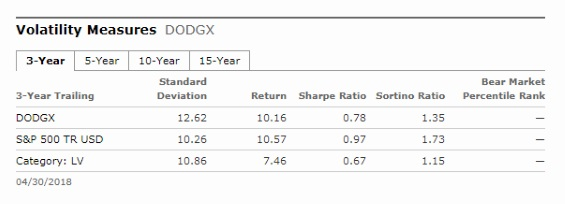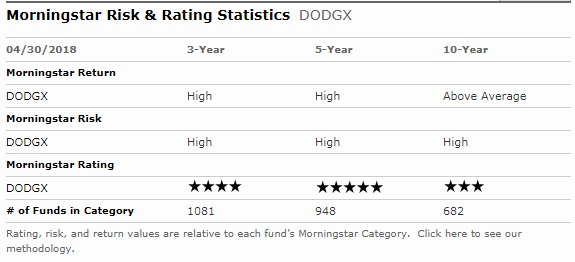Don't be intimidated by this technical-sounding performance measure. The concept is intuitive and the data is easy to interpret.

Q: Do risk-adjusted returns really matter? At the end of the day, isn't it actual returns that matter?

A: The first quarter of 2018 brought a refresher course in volatility after two very placid years of stock market gains. Now seems like a good time to reacquaint ourselves with volatility-adjusted return metrics.

If you'll allow me to get academic for a second, I'll refer to a concept in modern portfolio theory called the "efficient frontier." It's is the imaginary line that represents the combination of assets in a portfolio that will achieve the best possible return for the lowest level of risk, as measured by standard deviation. (If you envision risk on the x-axis, and return on the y-axis, the efficient frontier is an upward sloping line that represents the best combination of risk/return.) Every portfolio plotted below the line achieved an inferior return for the same amount of risk, or the same return with more risk.

Moving from the portfolio level to the individual fund level: There are metrics you can use to determine whether a particular mutual fund has rewarded investors for taking on a given level of risk. Suppose you have two mutual funds--an intermediate-term bond fund, and a large-cap blend equity fund. The bond fund earned a 5% annualized return over the trailing 10-year period, and the large-cap blend fund has gained 7% annualized. But the intermediate-bond fund's standard deviation was 3.33, while the large-blend fund's standard deviation was 14.99.

Standard deviation is a measure of how spread out the data are. In a normal distribution, 68% of the data will fall within one standard deviation of the mean; 95% will fall within two standard deviations; 99.7% within three, etc. At Morningstar, we collect all monthly total returns for the appropriate time period, and then all of the monthly standard deviations are annualized. For the large-cap blend fund, the mean return was 7%, and in more than 68% of cases, the returns were within one standard deviation (14.99)--meaning that they fell between –8% to 22%. That's a big range. When you see a higher standard deviation, you can imagine a shorter, fatter bell curve with thicker tails.

The bond fund, on the other hand, had a standard deviation of 3.33. In this case, you can imagine a higher, narrower bell curve with skinnier tails. Sixty-eight percent of the time, this fund's returns fell within one standard deviation of the mean return of 5%--in other words, 5% plus or minus 3.33--for a range of 1.67% to 8.33%.

Sharpe and Sortino Ratios
On a total return basis, the large-cap blend fund outperformed the bond fund. But what about when risk is taken into account? There are several ways to calculate risk-adjusted returns; perhaps the most widely known method is the Sharpe ratio. This is calculated by taking an asset's return minus a risk-free rate, which is the return you could get from investing in a virtually risk-free asset such as a 90-day T-bill (we'll use 1.8% in our example) and dividing by the standard deviation. The idea is to come up with a measure of return per unit of risk taken; the higher the resulting number the better the risk-adjusted return. Let's compute the Sharpe ratios for the two investments here:

Large-blend fund: (7% - 1.8%) / 14.99 = 0.35
Intermediate-term bond fund: (5% - 1.8%) / 3.33 = 0.96

When viewing the performance through the Sharpe ratio lens, it's really no contest; you get a much better return per unit of risk with the bond fund.

The Sharpe has a few drawbacks. For one, it treats upside and downside volatility as equally "risky," but in the world of investing, investors are more concerned with downside volatility than upside volatility.

That's where the Sortino ratio comes in. The calculation is set up very similarly to the Sharpe ratio--investment return minus risk-free rate in the numerator--but instead of using standard deviation in the denominator, it uses only the standard deviation of returns below the mean. Just like the Sharpe ratio, the higher the Sortino ratio the better, because that means that the investment is earning a higher return for each unit of downside risk it's taking on.

To find the Sharpe and Sortino ratios for funds on Morningstar.com, click on the "Ratings and Risk" tab on a fund's quote page and look under "Volatility Measures."